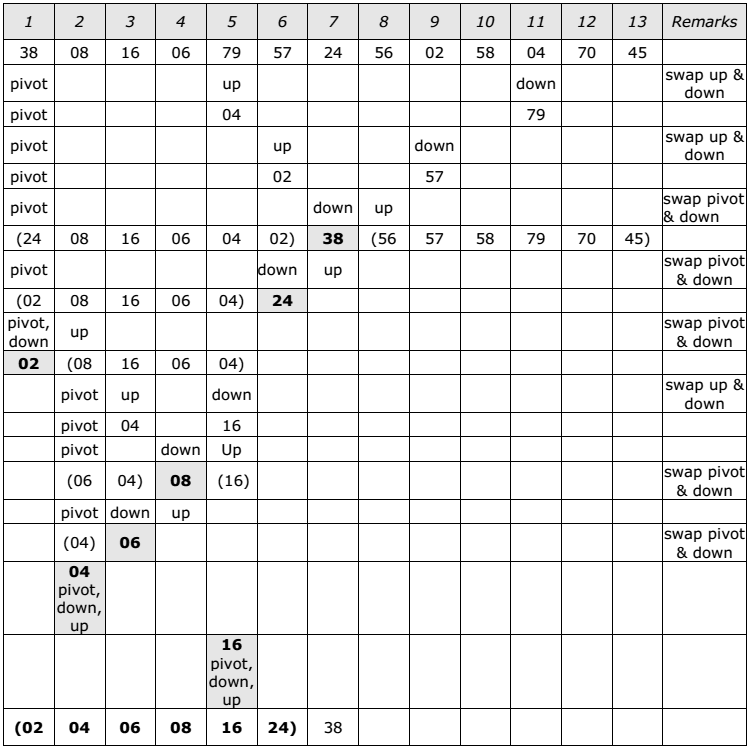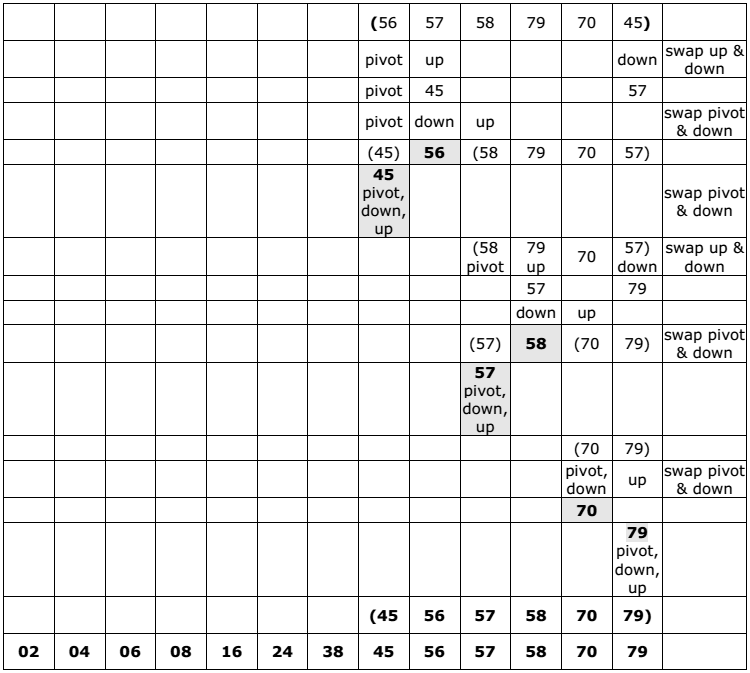The quick sort was invented by Prof. C. A. R. Hoare in the early 1960’s. It was one of the first most efficient sorting algorithms. It is an example of a class of algorithms that work by “divide and conquer” technique.

The quick sort algorithm partitions the original array by rearranging it into two groups. The first group contains those elements less than some arbitrary chosen value taken from the set, and the second group contains those elements greater than or equal to the chosen value. The chosen value is known as the pivot element. Once the array has been rearranged in this way with respect to the pivot, the same partitioning procedure is recursively applied to each of the two subsets. When all the subsets have been partitioned and rearranged, the original array is sorted.

The function partition() makes use of two pointers up and down which are moved toward each other in the following fashion:

1.  Repeatedly increase the pointer ‘up’ until a[up] >= pivot.
2. Repeatedly decrease the pointer ‘down’ until a[down] <= pivot.
3. If down > up, interchange a[down] with a[up]
4. Repeat the steps 1, 2 and 3 till the ‘up’ pointer crosses the ‘down’ pointer. If ‘up’ pointer crosses ‘down’ pointer, the position for pivot is found and place pivot element in ‘down’ pointer position.

The program uses a recursive function quicksort(). The algorithm of quick sort function sorts all elements in an array ‘a’ between positions ‘low’ and ‘high’.

1. It terminates when the condition low >= high is satisfied. This condition will be satisfied only when the array is completely sorted.
2. Here we choose the first element as the ‘pivot’. So, pivot = x[low]. Now it calls the partition function to find the proper position j of the element x[low] i.e. pivot. Then we will have two sub-arrays x[low], x[low+1], . . . . . . x[j-1] and x[j+1], x[j+2], . . . x[high].
3. It calls itself recursively to sort the left sub-array x[low], x[low+1], . . . . . . . x[j-1] between positions low and j-1 (where j is returned by the partition function).
4. It calls itself recursively to sort the right sub-array x[j+1], x[j+2], . . x[high] between positions j+1 and high.

The time complexity of quick sort algorithm is of O(n log n).

Example:
Select first element as the pivot element. Move ‘up’ pointer from left to right in search
of an element larger than pivot. Move the ‘down’ pointer from right to left in search of
an element smaller than pivot. If such elements are found, the elements are swapped.
This process continues till the ‘up’ pointer crosses the ‘down’ pointer. If ‘up’ pointer
crosses ‘down’ pointer, the position for pivot is found and interchange pivot and
element at ‘down’ position.
Let us consider the following example with 13 elements to analyze quick sort:You may be interested in:
Data Structures and Algorithms – MCQs.
Data Structures and Algorithms Online Tests